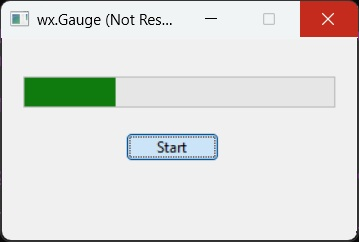# wxPython - Guage Class

Progressbar control in wxPython is called Gauge. Wx.Gauge class object shows a vertical or horizontal bar, which graphically shows incrementing quantity. It is typically used to demonstrate progression of a process like copying files or installing a software.

Wx.Gauge control can be used in determinate as well as indeterminate mode. When the time required to complete any operation can be fairly accurately determined, the gauge progress bar shows the percentage of completed task. However, in indeterminate mode, it only indicates that the process is ongoing.

In determinate mode, the progress position is updated periodically. In indeterminate mode, calling Pulse() function will update the progress bar.

Parameters required by Wx.Gauge class constructor is −

```wx.Gauge(parent, id, range, pos, size, style)
```

The range parameter sets the maximum value for the gauge. In indeterminate mode, this parameter is ignored.

The possible style parameters for Gauge class are −

S.N. Parameters & Description
1

wx.GA_HORIZONTAL

The horizontal layout of the progress bar

2

wx.GA_VERTICAL

The vertical layout of the progress bar

3

wx.GA_SMOOTH

Smooths progress bar with one pixel wide update step

4

wx.GA_TEXT

Displays the current value in percent

Some of the important methods of this class are listed in the following table −

S.N. Methods & Description
1

GetRange()

Returns the maximum value of the gauge

2

SetRange()

Sets the maximum value for the gauge

3

GetValue()

Returns the current value of the gauge

4

SetValue()

Sets the current value programmatically

5

Pulse()

Switches the gauge to indeterminate mode

## Example

In the following example, a horizontal Gauge object is added in the vertical box sizer of panel.

```self.gauge = wx.Gauge(pnl, range = 20, size = (250, 25), style = wx.GA_HORIZONTAL)
```

There is also a button whose click event is associated with a handler function.

```self.btn1 = wx.Button(pnl, label = "Start")
self.Bind(wx.EVT_BUTTON, self.OnStart, self.btn1)
```

The handler function OnStart() updates the progress of gauge after every second.

```def OnStart(self, e):
while True:
time.sleep(1);
self.count = self.count + 1
self.gauge.SetValue(self.count)

if self.count >= 20:
print "end"
return
```

The complete code for the example is as follows −

```import wx
import time
class Mywin(wx.Frame):

def __init__(self, parent, title):
super(Mywin, self).__init__(parent, title = title,size = (300,200))
self.InitUI()

def InitUI(self):
self.count = 0
pnl = wx.Panel(self)
vbox = wx.BoxSizer(wx.VERTICAL)

hbox1 = wx.BoxSizer(wx.HORIZONTAL)
hbox2 = wx.BoxSizer(wx.HORIZONTAL)

self.gauge = wx.Gauge(pnl, range = 20, size = (250, 25), style =  wx.GA_HORIZONTAL)
self.btn1 = wx.Button(pnl, label = "Start")
self.Bind(wx.EVT_BUTTON, self.OnStart, self.btn1)

hbox1.Add(self.gauge, proportion = 1, flag = wx.ALIGN_CENTRE)
hbox2.Add(self.btn1, proportion = 1, flag = wx.RIGHT, border = 10)

vbox.Add(hbox2, proportion = 1, flag = wx.ALIGN_CENTRE)
pnl.SetSizer(vbox)

self.SetSize((300, 200))
self.Centre()
self.Show(True)

def OnStart(self, e):
while True:
time.sleep(1);
self.count = self.count + 1
self.gauge.SetValue(self.count)

if self.count >= 20:
print "end"
return

ex = wx.App()
Mywin(None,'wx.Gauge')
ex.MainLoop()
```

The above code produces the following output −wxpython_major_classes.htm